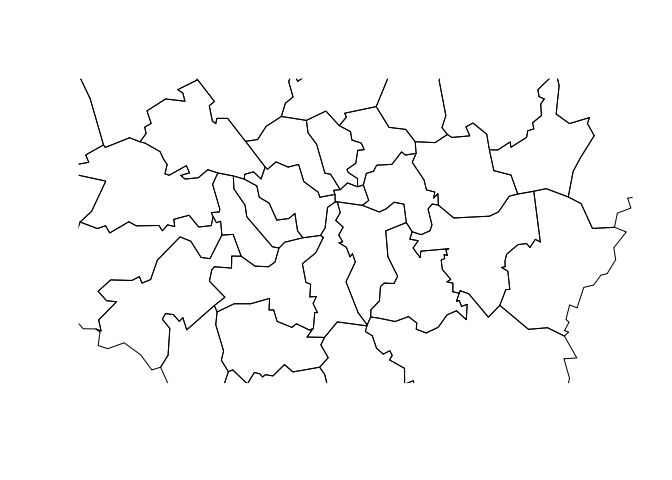Want to share your content on R-bloggers? click here if you have a blog, or here if you don't.

This miniature vignette shows how to clip spatial data based on different spatial objects in R and a ‘bounding box’. Spatial overlays are common in GIS applications and R users are fortunate that the clipping and spatial subsetting functions are mature and fairly fast. We’ll also write a new function called `gClip()`, that will make clipping by bounding boxes easier.To start with let’s load some data. I’m working in the root directory of the Creating-maps-in-R github repository, so there are some spatial datasets available to play with:

```setwd("../")
library(rgdal)

## OGR data source with driver: ESRI Shapefile
## Source: "data", layer: "lnd-stns"
## with 2532 features and 27 fields
## Feature type: wkbPoint with 2 dimensions

## OGR data source with driver: ESRI Shapefile
## Source: "data", layer: "london_sport"
## with 33 features and 4 fields
## Feature type: wkbPolygon with 2 dimensions```

## The wonder of spatial subsetting in R

Now, it’s easy to subset spatial data in R, using the same incredibly concise square bracket `[]` notation as R uses for non spatial data. To re-confirm how this works on non-spatial data, here’s a mini example:

```M <- matrix(1:10, ncol = 5)
M[2, 3:5]

##   6  8 10```

The above code creates a matrix with 5 columns and 2 rows: the `[2, 3:5]` part takes the subset of `M` corresponding to 3rd to 5th columns in the second row.

Subsetting spatial data works in exactly the same way: note that `zones` are far more extensive than the `stations` points. (We have to change the projection of `stations` before plotting, so the objects are on the same coordinate reference system.)

```stations <- spTransform(stations, CRS(proj4string(zones))) # transform CRS
plot(zones)
points(stations)```So how do we select only points that are are within the zones of London? Believe it or not, it’s as simple as subsetting the matrix `M` above. This is an amazingly concise and convenient way of spatial subsetting that was added to R at some point between versions 1 and 2 of Applied Spatial Data Analysis with R. In the earlier (2008) book, one had to use `overlay(x, y)` just to get the selection, and then another line of code was required to actually make the subset. Now things are much simpler. As Bivand et al. put it in the latest edtion (p. 131), “the selection syntax for features was extended such that it understands:”

`stations_subset <- stations[zones, ]`

This is so amazing and intuitive, whoever invented it should be given a medal!! Despite this simplicity, it seems many R users who I’ve taught spatial functions to are unaware of `[]`’s spatial extension. So spread the word (and if anyone knows of the history of this innovation, please let us know). Now we have a sample of all stations zones: progress.

```plot(zones)
points(stations_subset)```## Clipping by a bounding box

But what if we want to clip the polygons data, based on a bounding box? To start with, let’s look at and modify the existing bounding box for the zones, making it half the size:

```b <- bbox(zones)
b[1, ] <- (b[1, ] - mean(b[1, ])) * 0.5 + mean(b[1, ])
b[2, ] <- (b[2, ] - mean(b[2, ])) * 0.5 + mean(b[2, ])
b <- bbox(t(b))
plot(zones, xlim = b[1, ], ylim = b[2, ])```Now, to clip this area, we can use a custom function, which I’ve called `gClip`, following the rgeos function naming convention (this was inspired by an online answer that didn’t work for me):

```library(raster)
library(rgeos)

## rgeos version: 0.3-5, (SVN revision 447)
##  GEOS runtime version: 3.4.2-CAPI-1.8.2 r3921
##  Polygon checking: TRUE

gClip <- function(shp, bb){
if(class(bb) == "matrix") b_poly <- as(extent(as.vector(t(bb))), "SpatialPolygons")
else b_poly <- as(extent(bb), "SpatialPolygons")
gIntersection(shp, b_poly, byid = T)
}

zones_clipped <- gClip(zones, b)

## Warning: spgeom1 and spgeom2 have different proj4 strings

plot(zones_clipped)```Note that due to the `if` statements in `gClip`’s body, it can handle almost any spatial data input, and still work. Let’s clip to the borough of Westminster, one of London’s better known boroughs:

```westminster <- zones[grep("West", zones\$name),]
zones_clipped_w <- gClip(zones, westminster)

## Warning: spgeom1 and spgeom2 have different proj4 strings

plot(zones_clipped_w); plot(westminster, col = "red", add = T)```## Conclusion

There are many more spatial tips available from the Introduction to visualising
spatial data in
R

tutorial that James Cheshire and I are maintaining. The source code of this post can also be viewed online as just one of a series of vignettes to showcase some of R’s impressive spatial capabilities.